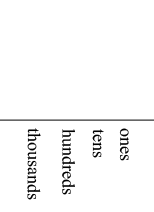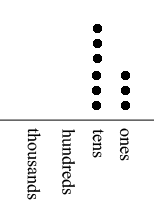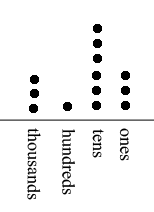SEARCH HOMEMath Central Quandaries & QueriesQuestion from Kristin, a parent: Make a place value drawing of a number that has double the number of tens as ones and three times the number of thousands as hundreds write the number.Hi Kristin,

I have not heard the term "place value drawing" so I did a Google search and found a number of such drawings. I am not sure what your child has seen in class so you might have to change the format. This is the format I am going to use and I am going to use dots to indicate the number of ones, tens, hundreds, and thousands.It's up to me to decide how many dots I put above the word ones and I am going to choose. three. Since the number of tens is double the number of ones the number of tens is six. Thus so far I haveWhen doing this with your daughter (?) I would have her choose the number of ones an deduce the number of tens. If she chooses five or more ones it won't work as each of the ones, tens, hundreds, and thousands has to be a digit. That is 0 to 9. It is legitimate to choose zero as the number of ones and then zero would also be the number of tens.

Now I get to choose again and I am going to choose one as the number of hundreds which forces the number of thousands to be three times one or three. Thus my completed place value drawing isNotice that the hundreds place value can not be larger than three or the thousands place would not be a digit. In this case I would not choose the hundreds place to be zero since I would then only get a two digit number. The number I get is 3163.

There are quite a few numbers you can obtain this way and I suggest that after your daughter get one of them you have her find another by using different ones and hundreds values.

I hope this helps,
PennyMath Central is supported by the University of Regina and the Imperial Oil Foundation.